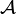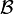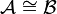# Isomorphism facts for kids

Kids Encyclopedia Facts

In mathematics (particularly in abstract algebra), two mathematical structures are isomorphic when they are the same in some sense. More specifically, an isomorphism is a function between two structures that preserves the relationships between the parts (see https://en.wikipedia.org/wiki/Isomorphism#Examples). To indicate isomorphism between two structures$\mathcal{A}$ and$\mathcal{B}$, one often writes$\mathcal{A} \cong \mathcal{B}$.

Using the language of category theory, this means that morphisms map to morphisms without breaking composition. An isomorphism is also a homomorphism that is one-to-one.

As an example, one can consider the operation of adding integers Z. The doubling function φ(x) = 2x maps elements of Z to elements of the even integers 2Z. Since φ(a+b) = 2(a+b) = 2a+2b = φ(a)+φ(b), adding in Z is structurally identical as adding in 2Z (which makes this an example of isomorphism).

## Related pagesIn Spanish: Isomorfismo para niños